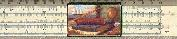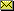# Base Eight

### And other math for people who are missing fingers Copyright (c) 1998 by Kenny Felder

This paper is about a whole new way to count.

Now, you may be wondering why anyone would bother with a whole new way to count, when the old way seems to work perfectly well. But I'm going to ask you to hold on to that question for the moment, and instead ask yourself something different: what's so special about the number ten?

You may never have asked yourself that. In our system of counting, everything changes after the number 9. You suddenly have to start all over and add another digit, and write "one-oh" (10). Sure, we call it "ten," but we write it "one-oh." Why do we do that? Think about that for a minute, and try to figure out the reason.

Trick question! There isn't any great reason. It's probably because we have ten fingers. So our ancestors had to start over when they ran out of fingers, and then again start over when they ran out of toes, and so we all got into the habit of starting over every tenth thing we counted. It didn't have to be that way. You can just as easily base a counting system on the number eight, or the number twelve, or just about any number you want. And in fact—getting back to my original question, of why anyone would bother—we do use alternative counting systems in some cases, such as in computers.

So here's what I'm going to do in this paper. First, I'm going to describe our normal ("base ten") counting system. You won't learn anything terribly new, but please don't skip that section, because it will present the ideas in a way that you may not have thought about them before. Then I'm going to go on to explain "base eight" counting. If you follow both sections, you will hopefully realize that they are both built on exactly the same principles, so once you can do one, you can do the other. Then at the end we'll test that knowlege, by asking you to do base sixteen!

Ready? Here we go, starting with...

### Base Ten, Also Known as "Decimal" Counting

Our normal counting system is called "base ten" because we have exactly ten symbols to work with:

0 1 2 3 4 5 6 7 8 9

Out of those ten symbols, we have to be able to represent every possible number from 1 all the way up as high as we can go. How do we do that? Well, we start by listing all the symbols after the "zero."

1
2
3
4
5
6
7
8
9

When we get to that point, we're out of symbols. So what do we do? We go all the way down to zero, and add a one to our left: we write "one-zero" (10). This strange two-number pair means "the number after nine," or what we generally call "ten."

Now we start counting on the right again: one-one, one-two, one-three, and so on. (11, 12, 13...) Soon we hit one-nine (19) and we run out of digits again, so we have to increment on the left: two-zero, or 20. You see what I mean? Every tenth number, we start over again.

This system works great until we get to 99, and then we can't increment the left-hand digit any more. So we move to the left again and write one-zero-zero (100)...and so on.

This is all very familiar, I expect. But it's good to review it and get it clear in your mind. When I write the decimal number 1235 in this system, what do I mean? I mean 5 "ones", 3 "tens", 2 "hundreds", and 1 "thousand." Each step to the left involves a multiplication by ten—because we are in base ten. So if there were another digit to the left, we know it would count as "ten thousands" since that is ten times "thousands," and so on.

Please stop at this point and make sure everything I've just said makes perfect sense to you. It isn't enough to be able to count: you have to understand the theory in the way I've described it. If it all makes sense, the rest—believe it or not—should be pretty easy.

### Introducing Base Eight

So what if we had eight fingers, or for some other reason, we decided to start over every eighth number instead of every tenth? Then we would have "base eight" (also known as "octal") counting. In this system, there are eight symbols to work with:

0 1 2 3 4 5 6 7

We don't need an 8 or a 9 at all: out of just those eight symbols above, we are going to represent every possible number! So, we start by listing all the symbols after the "zero."

1
2
3
4
5
6
7

When we get to that point, we're out of symbols. So what do we do? We go all the way down to zero, and add a one to our left: we write "one-zero" (10). It means "the number that comes after seven," or what we normally call "eight." This is the key turning point in this paper, so make sure you're still with me: when I write "one-oh" (10) in base eight, I don't mean ten, I mean the number eight. The numbers in base eight look just like our normal numbers (except that they never use the symbols 8 or 9), but they don't mean the same things. You have to think in "eights" to understand them, just as you have to think in "tens" to understand our normal system.

Now we start counting on the right again: one-one, one-two, one-three, and so on. (11, 12, 13...) Soon we hit one-seven (17) and we run out of digits again, so we have to increment on the left: two-zero, or 20. Every eighth number, we start over again.

This system works great until we get to 77, and then we can't increment the left-hand digit any more. So we move to the left again and write one-zero-zero (100). It's important to remember again that this doesn't mean the same thing we normally call "one hundred" so it's best not to call it that: call it "one-zero-zero" and it will help keep things straight.

So, when I write the octal number 1235 what do I mean? I mean 5 "ones", 3 "eights", 2 "sixty-fours", and 1 "five-hundred-and-twelve." Each step to the left involves a multiplication by eight—because we are in base eight. So if there were another digit to the left, we know it would count as "four-thousand-and-ninety-sixes" since that is eight times "five-hundred-and-twelves," and so on.

If you've followed everything I've done so far, you have hopefully understood a few things. First, you understand that our normal system of counting is based on the principle that every tenth number, you start over and move to the left—because there are only ten symbols to work with. Second, you understand that there's nothing sacred about the number ten: base eight is exactly the same, except it happens to start over every eighth number, because it has eight symbols to work with. Once you can do base ten counting, there's nothing fundamentally different about base eight.

But you may still be wondering, what does 1235 base eight really mean? Well, I said above that it is 5 "ones", 3 "eights", 2 "sixty-fours", and 1 "five-hundred-and-twelve." If you add all that up,

5*1 + 3*8 + 2*64 + 1*512 = 669

So we can say "1235 base eight equals 669 base ten." Or, to put it another way, "when you write 1235 in base eight, you really mean the number six-hundred and sixty-nine." That's a kind of base-ten-centric way to put it, but hey, we're base-ten-centric people. And in any case, the point I'm making is that it's easy to convert base eight numbers into their base ten equivalents, once you understand the system.

What I've just done is give a lecture on "how to count" in base ten, and then repeat the exact same lecture for base eight.

Base sixteen, or "hexadecimal" notation, works exactly the same way, except that it starts with sixteen symbols:

0 1 2 3 4 5 6 7 8 9 A B C D E F

Just as base ten uses a few symbols that base eight doesn't need ("8" and "9"), base sixteen uses a few symbols that base ten doesn't need. These symbols are the first six alphabetic characters. So A represents the number we usually call "ten"; the highest single digit is F which represents our "fifteen." After that you have to start over.

What I would like to ask you to do, at this point, is to duplicate the exact "lecture" I gave above on base eight, except you do it in base sixteen. Start where I wrote "we start by listing" and go all the way to "1235 base eight = 669 base ten," but write everything in terms of base sixteen. Of course, the final answer (1235 base sixteen equals what in base ten?) will be different: work the problem yourself, then click here to check your answer.Gary and Kenny Felder's Math and Physics Help Home Page
`www.felderbooks.com/papers`Send comments or questions to the author